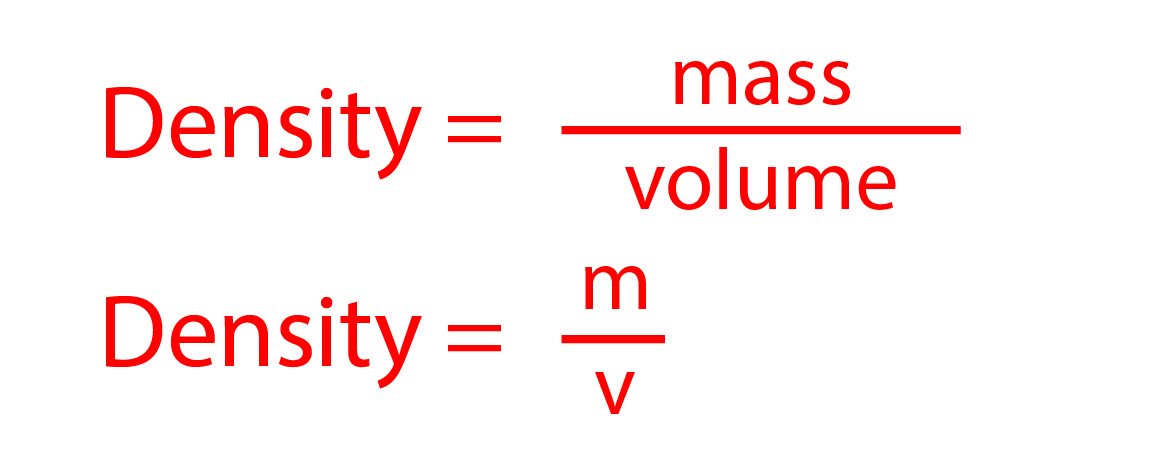# Density

Density is a measure of how tightly packed the molecules of a solid, liquid or gas are. The formula for calculating density is:The dense city (density) was so dense they built motorways over any vegetation.

NOTE:

m = mass in kilograms (kg)

V = volume in cubic metres (m)

D = Density in kilograms per metre cubed (kg//m^3)

Example 1

John wants to measure the density of a rock. He finds the volume with the apparatus shown below:The mass of the rock was measured as being 36g. What is the density of the rock?

Density=(mass)/(volume)

First convert everything to standard units

Mass of the rock = 36g = 0.036\ kg

Volume of rock =36cm^3-18cm^3=18cm^3=0.000018m^3

Density of the rock =0.036/0.000018=2000\ kg//m^3